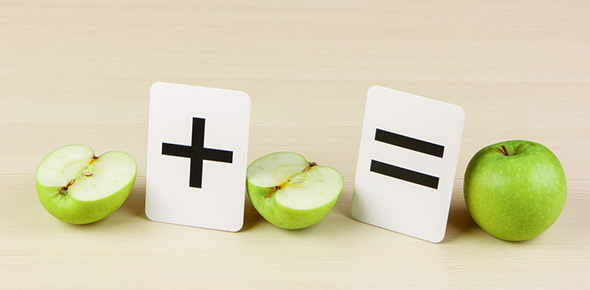# Fraction IT Quiz

10 Questions | Total Attempts: 51SettingsA quiz to test your knowledge and understanding of fraction names, number lines and terminology.

Related Topics
• 1.
How is this fraction written in words?
• A.

One-Third

• B.

A Third

• C.

A Half

• D.

One-fourth

• 2.
What fraction does this picture represent?
• A.

One-Fifth

• B.

One-Third

• C.

One-Half

• D.

Two-Thirds

• 3.
The numerator in the below fraction is which number? 1 or 4?
• 4.
The denominator in the below fraction is which number? 3 or 5?
• 5.
Which fraction would best describe the location of the red dot on the number line?
• A.

1/2

• B.

1/5

• C.

2/4

• D.

3/5

• 6.
This circle as a whole number has been sectioned in to how many equal parts?
• 7.
How many equal parts is this number line sectioned in to to make 1 whole?
• A.

2

• B.

4

• C.

1

• D.

3

• 8.
What fraction does this picture represent?
• A.

One-Third

• B.

1/5

• C.

Two-Quarters

• D.

2/3

• 9.
True or false.  All of the below fractions equal 1 whole number.
• A.

True

• B.

False